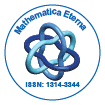Mathematica EternaOpen Access

ISSN: 1314-3344+32-466902147

Abstract

On a different kind of d-orthogonal polynomials that generalize the Laguerre polynomials

Serhan Varma and Fatma TaÃÂ¸sdelen

The purpose of this work is to give an another generalization of the Laguerre polynomials in the context of d-orthogonality by a generating function of a certain form. We derive the d-dimensional functional vector for which the d-orthogonality holds. Some properties of the obtained polynomials are determined: expilicit representation, relation with a known polynomial, a recurrence relation of order-(d + 1) and a differential equation of order-(d + 1).

Top